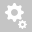##Recently viewed tickets# Concurrent Beam Stress due to Moving Load is Incorrect

Question:

Dear Midas,

I have performed a manual calculation on the beam stresses due to moving load but somehow, I unable to recreate the beam stress shown in Midas.
This problem is found when analysis the beam stress due to moving load but static load is fine, I can calculate the beam stress accurately.
I have attached my testing model in case you need it.Hello,

The moving load results are envelope of results.
For HA:max, the maximum results will displayed be for each component of force/stress for each element and it will be independent of each other.
For eg: for above case HA:kel (Max) if we look at My, then it is max bending moment-y occuring in element 2, similarly for Mz is max bending moment -z occurring in element 2. The max moment My and Mz value may or maynot occur simultaneously to one vehicle position.
So, it doesn't display concurrent beam results. It displays max/min results in the envelope of results formed by running the vehicle on the traffic lane.
So, stress shown here are max stress for each component. Similarly for the forces.
Thus, they can't be directly combined. They first need to be converted to static load using moving load tracer and stresses are to be verified using that static load results.
Video on forming load using moving load tracer is attached for reference.

Question
Or I can view its concurrent stress as shown below?As you can see that even if we enveloped all the concurrent beam stresses of element 2,
for example, Cb3, the maximum stress it experienced is 3.847MPa but
in HA KEL(max) from our first post, it displays as 2.547 MPa. Why?

Hello,

Before explaining the results, let me tell some key points,

1) Midas form separate influence line diagram for each component of force and stress. Therefore, separate influence line for axial stress, shear stress and cb1 to cb4 etc.

2) The vehicle is placed on influence line such that critical effect can be obtained. Thus, W is placed depending on the influence line.

3) Value of W in vehicle definition changes with length of the bridge span loaded.Now, when the results are viewed in beam stress table, the vehicle are placed on the corresponding influence line to obtain the critical results.But in "Result by Max- Value" table, the concurrent stresses are obtained.
So, if we observe the shear-y for HA KE: min, we observe the larger value for bend (z) and  cb3 value which is not correct value to take because

The vehicle is placed according to influence line of shear -y. The vehicle loaded length decreases and thus w value increases. Therefore, the higher value bend-z, bend+z etc. are obtained for that loaded vehicle position. Therefore, higher cb1 to cb4,

But in beam stress, results in cb3  are displayed are according to influence line of Cb3. For that, the loaded length is full span and thus separate results/ lower results are obtained due to lesser value of w.So, actually the span length being small is causing such disparity in results as w is highly changing for lower loaded lengths values.

Creation date: 6/8/2021 2:10 PM (jatin2010)      Updated: 6/8/2021 2:10 PM (jatin2010)